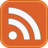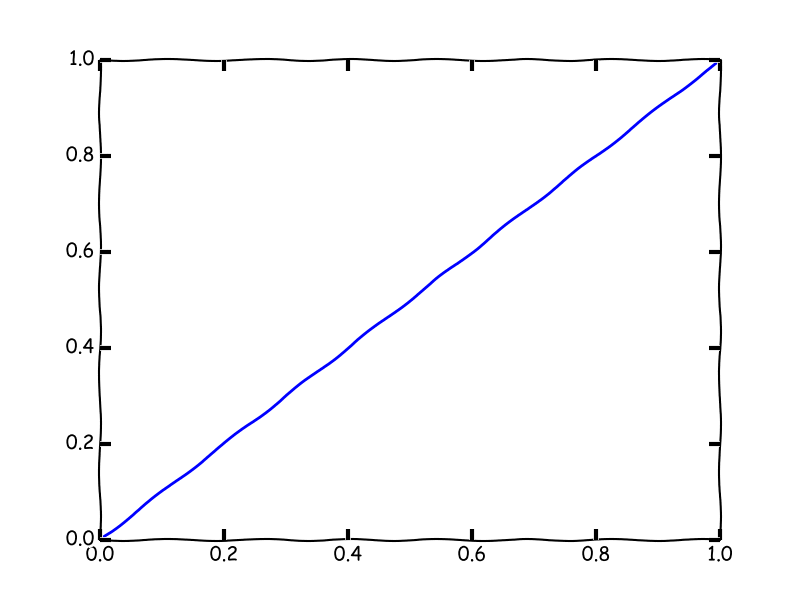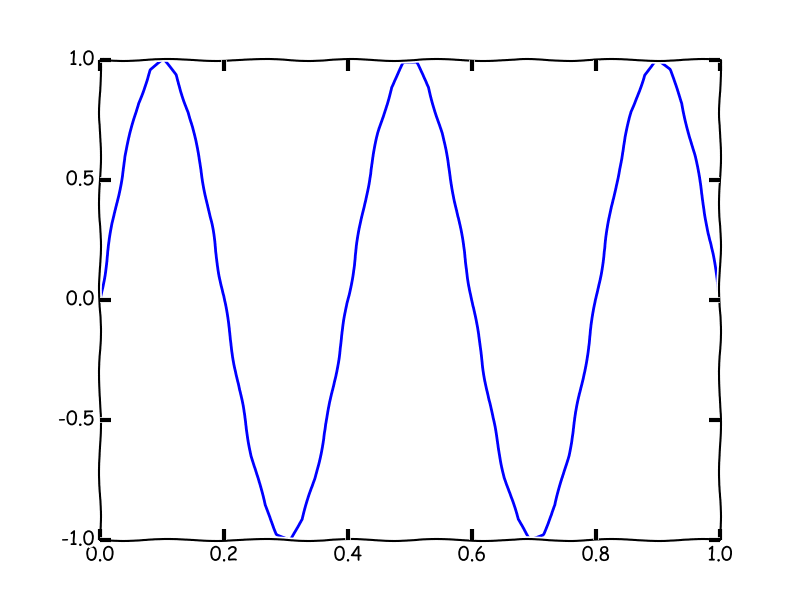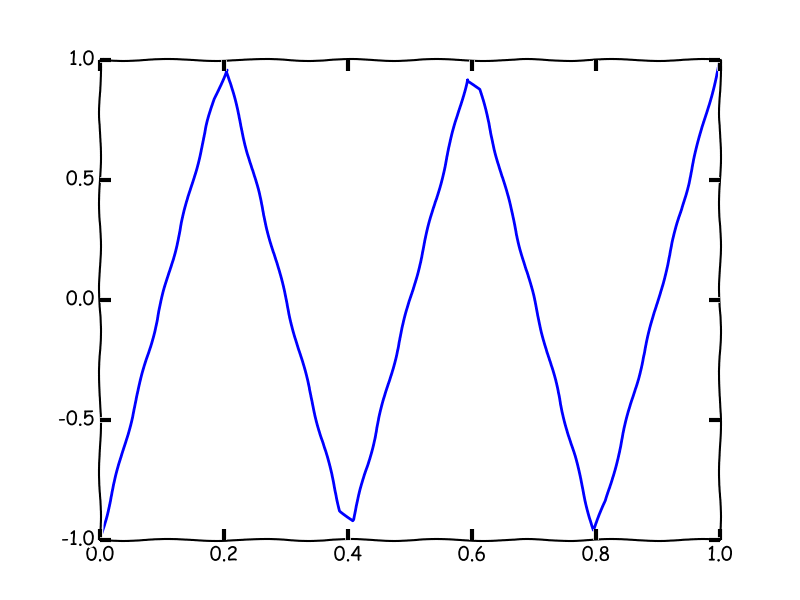# James Percival

## My Github Pages material

Last updated : 30th August 2018

# Teaching Page

## Finite Element Methods

Mathematical descriptions of the equations governing physical processes are often in the form of partial differential equations (PDEs). Variable values are functions of time and satisfy relationships between variables themselves and their higher partial derivatives. Some architypal examples :
• The heat equation, $$\frac{\partial T}{\partial t}=\kappa\frac{\partial^{2}T}{\partial x^{2}},$$
•• The wave equation, $$\frac{\partial^{2}a}{\partial t^{2}}=c^{2}\frac{\partial^{2}a}{\partial x^{2}},$$
•• The advection-diffusion equation $$\frac{\partial\tau}{\partial t}+u\frac{\partial\tau}{\partial x}=\kappa\frac{\partial^{2}\tau}{\partial^{2}x}.$$
•Frequently we would like to generate sufficiently accurate numerical solutions to these equations using a computer. However computers (and humans) possess only a finite amount of memory to store the results of their calculations, while even a simple a problem domain such as the real numbers between 0 and 1 provides an uncountably infinite number of locations for which variable data would be required. As such, it is necessary to discretize the problem down to operations on a finite dimensional space on which is tractable to perform calculations. There are a number of approaches aimed at achieving this. In this course we will discuss several approaches you may already be familiar with, then introduce the finite element method, which you may not.
Notes may be found here.